Courses

# Transportation & Assignment Mechanical Engineering Notes | EduRev

## Mechanical Engineering : Transportation & Assignment Mechanical Engineering Notes | EduRev

The document Transportation & Assignment Mechanical Engineering Notes | EduRev is a part of the Mechanical Engineering Course Industrial Engineering.
All you need of Mechanical Engineering at this link: Mechanical Engineering

Transportation

The transportation problem is a special type of linear programming problem where the objective is to minimize the cost of distributing a product from a number of sources or origins to a number of destinations. It is also sometimes called as Hitchcock problem.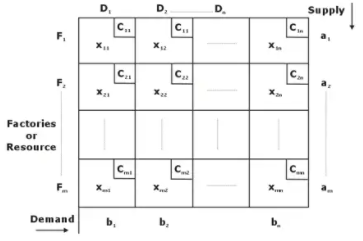Destinations

Total no. of cells = m × n
Total no. of feasible allocations = m + n – 1
Total no. of alternate solutions = nCm
The aim of transportation problem is to reduce the cost of transporting commodities from different suppliers to different destinations.

Mathematically, Minimize,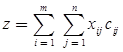Subject to the constraints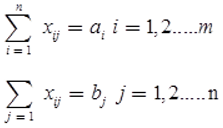Xij ≥ 0(for all i and j

Existence of Feasible Solution

A necessary and sufficient condition for the existence of a feasible solution to the general transportation problem is that
Total supply = Total demand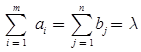Existence of Basic Feasible Solution

The number of basic variables of the general transportation problem at any stage of feasible solution must be (m + n – 1). Now degenerate basic feasible solution (a feasible solution) involving exactly (m + n – 1) positive variables is known as non-degenerate basic feasible solution. Otherwise it is said to be degenerate basic feasible. These allocations should be independent positions in case of non-degenerate basic feasible solutions.

Key Points

• Optimum Solution: A feasible solution is said to be optimal, if it minimizes the total transportation cost.
• Unbalance TP: If total supply is not equal to total demand, then it balance with dummy source or destination.

Solution of a Transportation Problem

• Step 1: Formulate problem as LPP.
• Step 2: Set up transportation table.
• Step 3: Examine total supply = total demand if not then introduce a dummy row or column having all its cost element as zero.
• Step 4: Find an initial basic feasible solution that must satisfy all the supply and demand conditions.
• Step 5: Examine the solution obtained in Step 4 for optimal i.e., examine whether an improved transportation schedule with lower cost is possible.
• Step 6: If the solution is not optimum modify the shipping schedule by including that unoccupied cell whose inclusion may result in an improved solution.
• Step 7: Repeat step 4 until no further improvement is possible.

To find the initial basic feasible solution there are three methods

• Northwest Corner Cell Method
• Row Minima method
• Column Minima method
• Least Call Cell Method
• Vogel’s Approximation Method (VAM).

1. North-west corner Rule

• Set up the balanced transportation problem.
• Select the North west corner cell in the cost matrix, allocate as much as possible to the cell based on supply and demand.
• In the allocated cell’s row or column is saturated/exhausted then delete the corresponding row column.
• Repeat the above process till the entire allocation is completed.

2. Row-minima method

• 1st → min cost move along row
• Rest same as above method.

3. Column minima method

• 1st → min cost move along column.
• Rest same as above method.

4. Least cost method or Method of matrix minima

• Set up the balanced transportation problem
• Consider the least unit cost cell in the matrix for allocation. If tie occurs, then select the highest allocation possible cell for the allocation.
• If the allocated cell’s row or column is saturated/exhausted then delete the corresponding row or column.
• Repeat the above process till the entire allocation is completed.

5. Vogel’s Approximation method (VAM) or Unit cost penalty

• In this method we take the difference between smallest and 2nd smallest element in each row and column and write them below the respective row and column.
• Select the highest individual difference & max. possible allocation is done in thin cost cell of selected row or column.
• The row or column whose requirement becomes zero is striked-off show that it can’t be considered again continuing the similar manner until all the allocations are done.
• In this method we allocate one unit to an unallocated empty cell and compute the effect on the cost of matrix it is an into and trial method in which chances of making error are more.

6. Test for Optimality in Transportation Problems

• Determine the net evaluations for non-basic variables (unoccupied cells) (u, v method).
• Choosing that net evaluation which may improve the current basic feasible solution.
• Determining the current occupied cell which leave the basis and repeating step 1 through step 2 ultimate an optimum solution is attained.

Assignment Model

The assignment problem is a special case of the transportation problem in which the objective is to assign a number of resources to the equal number of activities at a minimum cost (or maximum profit).
Assignment, problem is complete degenerate form of transportation problem. That means exactly one occupied cell in each row and each column of the transportation table i.e., only n occupied cells in place of the required (n + n - 1) = (2n - 1)

Conditions to be satisfied for Assignment model

m = n
xij = 0 or 1
all ai = 1
all bj = 1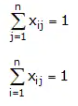Hungarian Method for Solving Assignment Problem

The Hungarian method of assignment provides us with an efficient method of finding the optimal solution without having to make a-direct comparison of every solution. It works on the principle of reducing the given cost matrix to a matrix of opportunity costs.
Opportunity cost show the relative penalties associated with assigning resources to an activity as opposed to making the best or least cost assignment. If we can reduce the cost matrix to the extent of having at least one zero in each row and column, it will be possible to make optimal assignment.

The Hungarian method can be summarized in the following steps:

• Step 1: Determine the cost table from the given problem.
• Step 2: In case of number of resources is not equal to number of destinations, add a dummy source or dummy destinations so that cost table becomes a square matrix. The cost entries of dummy sources/destinations are always zero.
• Step 3: Locate the smallest element in each row of the given cost matrix and then subtract the same from each element of that row.
• Step 4: In the reduced matrix obtained in Step 3. Locate the smallest element of each column and then subtract the same from each element of that row.
• Step 5: Examine the rows successively until a row with single zero is found. () this zero and cross all other zero. do it same for all rows.
- Repeat the procedure for each column.
- In case of two zero in same row or column choose arbitrary any one of these zero and cross off other zeros.
- Repeat (a) through (c) above successively until the chain of assigning () or cross (x) ends.
• Step 6: If number of assignments () is equal to n an optimum solution is reached. If number of () <n then go to next step
• Step 7: Draw the minimum number of horizontal or vertical lines to cover all the zeros of the reduced matrix.
• Step 8: Developed the new revised cost matrix.
• Step 9: Go to Step 6 and repeat the procedure until an optimum solution is attained.
Offer running on EduRev: Apply code STAYHOME200 to get INR 200 off on our premium plan EduRev Infinity!

## Industrial Engineering

33 videos|30 docs|32 tests

,

,

,

,

,

,

,

,

,

,

,

,

,

,

,

,

,

,

,

,

,

;